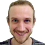## Wednesday, June 10, 2009

### Probability of a graph being connected...

I ran across the following question in a problem I'm working on, and thought I'd ping the collective wisdom of the blog-reader-o-sphere.
Given an undirected graph G with (possibly different) probabilities on edges, consider the usual random process where we pick each edge independently, but with probability p_e (this is the difference from the standard erdos-renyi model).

What is the probability that the resulting graph is connected ?

This seems possibly to have something to do with the spanning tree polytope, but it seems quite nontrivial. In general, I'm given a subset of vertices S in this graph, and want to ask for the probability that S forms a connected component. Making sure that S is disconnected from the rest of the graph is easy, but then the remainder of the probability comes from the answer to the above question.

I should add that the subset connectivity question is trivial if G is a tree: it's the higher connectivity case that makes things harder.

p.s More SoCG posts should be on their way in the next few days after I return.

1.Check Karger's paper.
http://people.csail.mit.edu/karger/Papers/reliability-sirev.ps

2.The following paper of Noga Alon considers this question.

http://www.cs.tau.ac.il/~nogaa/PDFS/connect.pdf

In summary, the graph will be connected whp if min-cut under p is at least clog n for some constant c and the bound is tight upto constants.

Mohit

3.Does this assume that each edge has a different probability of existence?

Would the work of Newman (on graphs with arbitrary degree distributions) help in this respect?

4.A good problem- you would think something like the matrix-tree theorem would solve it.

I apologize if I misread your details or intent (weather you were interested in specifics graphs or asymptotics) as my comment is only appropriate if we are talking about the exact same problem.

If you re-phrase graph connectivity as network reliability problem this problem is known to be hard ( #P complete: Provan and Ball " The complexity of counting cuts and of computing the probability that a graph is connected" SIAM J. Comput. 12/4, (1983), 777-788). The survey I was looking at was "A Note on the Complexity of Network Reliability Problems" Hans L. Bodlaender and Thomas Wolle.

5.Thanks all for the incredibly fast and useful comments. Chandra and John, your references were dead on: the Provan and Bell Paper (referenced in the Karger paper) shows that the problem is #P-complete, and is even hard to approximate.

Mohit, thanks for the pointer to Noga Alon's paper.

Panos, yes I was interested in the case of differing probabilities.

6.Is the probability that any particular two vertices are connected a constant?

In that case, I solved this problem in the past.

Define:

- P_connected(N): probability that a graph with N vertices is connected (given that the probability of any two vertices being connected is a constant P_e).

- P_disconnected(N) = 1-P_connected(N)

Now, you can write a recurrence that expresses P_connected(N) using P_connected(N-1) and P_disconnected(N-1). It should be straightforward, but very easy to mess up the details.

Now, you have a formula to compute P_connected(N+1) from P_connected(N). Start with the base case P_connected(1) = 1, and build up to the desired N.

7.I don't think it is that easy, Igor. Your recurrence should have about the Bell number of terms..

8.This topic is a little old, but I hope some of you will still read my question. I also need to find the probability of a graph to be connected except that this graph is not random but defined as follows:

Let (x1, x2, ..., xn) be a sequence of points in R^n. Define graph G with edge (i,j) iff ||xi-xj|| <= R with R a given constant.

I assume one possible way would be to compute the probability of existence of an edge and then use the results from connectivity on random graphs. I'm hoping there may be a more ad hoc way to proceed.